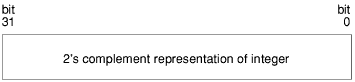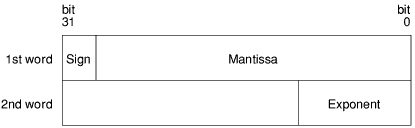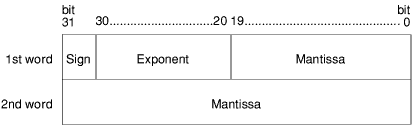# Appendix A - Numeric implementation

Before you can perform any arithmetic operations, you need to know how the computer handles numbers, and what limitations there are on their use.

This appendix describes the different types of numbers you can use with BBC BASIC, tells you how they are stored and manipulated, and explains what limitations this places on your programs.

#### Numeric types

You can use the following numeric types with BBC BASIC:

##### Integers

These are whole numbers, which can be represented exactly by the computer, for example:

1
2
1024

##### Floating point numbers

These are real numbers expressed as a decimal fraction, for example:

1.3
123.45
1.2345E2

##### Fixed point numbers

These are real numbers expressed as a decimal fraction, but with a fixed number of places after the decimal point. For example:

1.3333
1.2346
123.4568

are fixed point numbers accurate to four decimal places.

The most important factor governing numeric types is the amount of memory used to store them. For the purposes of this description, we will only consider integers and floating point numbers.

BASIC VI uses the following storage sizes for numeric types:

Numeric type Storage size
Integers 4 bytes (32 bits)
Floating point numbers 8 bytes (64 bits)

Remember that BASIC V only supports integers and 5-byte reals (we shall use the term n-byte reals to mean n-byte floating point numbers). The following figures show how the storage for each numeric type is organised.#### Effects of storage size

The storage size of a numeric type affects the following things:

• the speed with which numbers of that type are processed by the computer;
• the amount of memory left for your program;
• the range of numbers of that type which can be represented by the computer;
• the accuracy with which numbers of that type can be represented by the computer.

For example, integers occupy less space than real numbers, and are handled much more quickly. 8-byte reals use more memory than 5-byte reals, and are therefore more accurate. The computer can represent larger numbers in the 8-byte format.

The effect on memory usage is very important if, for instance, your program uses arrays of real numbers. Consider an array with 100 elements in, each element being a 5-byte real. This will occupy 500 bytes of memory, whereas it would occupy 800 bytes if the elements were 8-byte reals. This is a trivial example, but the effects can become severely limiting if you use very large arrays.

The following two subsections explain range and accuracy of representation in more detail.

##### Range

The greater the storage size of a given numeric type, the greater the range of numbers of that type that the computer can represent. For instance, integers are stored in 4 bytes or 32 bits. The maximum positive integer that the computer can represent is given by

2 ^ (32 - 1)

which means 2 raised to the power of 31, and is equal to 2147483647.

The maximum positive real number that the computer can represent depends on which type of real number you specify. For instance, the maximum positive 5-byte real that the computer can represent is

1.7 x 1038

##### Accuracy

The accuracy of a number is determined by how many significant figures of the number that the computer can show. The computer can show all the significant figures in an integer (as long as it is within the representable range). However, it must lose some of the significant figures of a floating point number.

For instance, the value of PI shown to three significant figures is 3.14. Shown to six significant figures, it is 3.14159. BASIC VI can show up to 17 significant figures of a floating point number, but this does not mean it is completely accurate. PI has an infinite number of digits after the decimal point, and so the computer can only print an approximation to it, by chopping off the trailing digits.

The table below summarises the numerical representation of BBC BASIC.

Range Accuracy Stored in
Integers -2147483648 to 2147483647 absolute 4 bytes
5-byte reals ±1.7x1038 to ±1.5x10-39 9 sig figs 5 bytes
8-byte reals ±1.7x10308 to ±1.5x10-323 17 sig figs 8 bytes

The rest of this appendix explains the two methods used by BASIC VI for implementing 8-byte floating point arithmetic to IEEE standard 754. BASIC V only employs one of these methods, and does perform its 5-byte arithmetic to the IEEE standard.

#### What is floating point arithmetic?

Floating point arithmetic is the process by which real numbers are manipulated, as a result of your instructions to the computer. For example, a computer cannot add two numbers together in the way we can. It must first convert the numbers into binary form, and then add them using Boolean operations.

Every arithmetic operation can be reduced, at the lowest level, to a group of Boolean operations. It is more convenient, however, to represent these groups by a set of mnemonics, called the floating point instruction set. The BBC BASIC floating point instruction set is given in full in the RISC OS Programmer's Reference Manual.

#### Implementation

BBC BASIC VI uses two methods to implement floating point arithmetic. They are as follows:

• software implementation, using a floating point emulator (FPE)
• hardware implementation, using an optional floating point coprocessor.

The advantage of hardware implementation is that it is much faster.

When you instruct the computer to add two real numbers A and B together, the following sequence of events takes place:

1. The BASIC interpreter stores the numbers in floating point format.
2. The ARM processor scans the list of operations it can perform. It cannot perform floating point operations itself, so one of the following two things can happen:
• The instruction is performed by the floating point coprocessor (if fitted).
• The instruction is performed by the floating point emulator.
3. The interpreter produces machine code instructions, telling the ARM microprocessor that the floating point numbers A and B are to be added together using a floating point add instruction (ADF).
4. The ARM processor stores A and B in its internal floating point registers.

##### Floating point emulator

The floating point emulator is a software module that provides floating point support. It emulates a hardware floating point coprocessor. It is this module that provides the floating point instruction set, extending the existing instruction set of the ARM processor.

You cannot use floating point instructions directly, as the BASIC interpreter does not understand them. However, you can include them in an assembly language module which is called from your program. The description of the CALL statement (in the Keywords chapter) explains this.

##### Floating point coprocessor

The floating point coprocessor is an optional hardware device that performs floating point arithmetic to IEEE standard 754. The coprocessor only directly supports a subset of the floating point instruction set. If a particular instruction is not supported by the coprocessor, it is performed by the emulator instead.

It does not matter to your program whether a coprocessor is present or not. The user interface ensures that programs run in exactly the same way in either case. The only difference you will see is in the speed at which your program runs.

Floating point instructions are performed much faster in hardware, although the actual improvement in performance depends on what equipment you are using, and which processor you have.